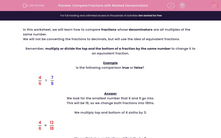# Compare Fractions with Related Denominators

In this worksheet, students must compare fractions whose denominators are multiples of the same number.Key stage:  KS 2

Curriculum topic:   Maths and Numerical Reasoning

Curriculum subtopic:   Fractions

Popular topics:   Equivalent Fractions worksheets

Difficulty level:#### Worksheet Overview

In this worksheet, we will learn how to compare fractions whose denominators are all multiples of the same number.

We will not be converting the fractions to decimals, but will use the idea of equivalent fractions.

Remember, multiply or divide the top and the bottom of a fraction by the same number to change it to an equivalent fraction.

Example

Is the following comparison true or false

 4 > 7 6 9

We look for the smallest number that 6 and 9 go into.

This will be 18, so we change both fractions into 18ths.

We multiply top and bottom of 4 sixths by 3:

 4 = 12 6 18

We multiply top and bottom of 7 ninths by 2:

 7 = 14 9 18

Now

 12 < 14 18 18

So the statement

 4 > 7 6 9

is FALSE.

### What is EdPlace?

We're your National Curriculum aligned online education content provider helping each child succeed in English, maths and science from year 1 to GCSE. With an EdPlace account you’ll be able to track and measure progress, helping each child achieve their best. We build confidence and attainment by personalising each child’s learning at a level that suits them.

Get started